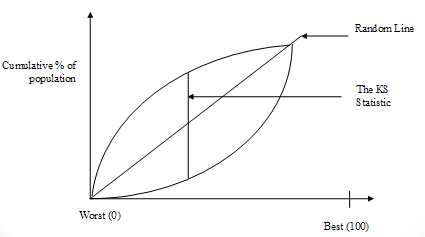# SAS : Calculating KS Statistics

In predictive modeling, it is very important to check whether the model is able to distinguish between events and non-events. There is a performance statistics called "Kolmogorov-Smirnov" (KS) statistics which measures the discriminatory power of a model. It is a very popular metrics in credit risk modeling.

## What is Kolmogorov-Smirnov (KS) Statistics?

It looks at maximum difference between distribution of cumulative events and cumulative non-events. First step is to split predicted probability into 10 parts (decile) and then compute the cumulative % of events and non-events in each decile and check the decile where difference is maximum (as shown in the image below.)
In the image below, KS is 57.8% and it is at third decile.Calculating KS Statistic
KS curve is shown below. It is drawn by plotting Cumulative % of population. Better the KS, better the model.KS Statistics

There is another way of calculating KS Statistics :

Compute KS Two Sample Test with proc npar1way. It generates the difference metrics. See the SAS code in the next section.

## Calculating KS Statistics with SAS

Let's prepare fake data for dependent variable
```data full;
do i=1 to 1000;
x=rannor(12342);
p=1/(1+exp(-(-3.35+2*x)));
y2=ranbin(98435,1,p);
drop i;
output;
end;
run;
```
KS Statistics with PROC NPAR1WAY
```proc logistic data= full;
model y2(event="1")=x;
output out=out2 p= pred;
run;

Proc npar1way data=out2 edf;
class y2;
var pred;
run;
```KS Output

The D statistic (highlighted in the image above) is the metrics that is used to report KS score. DO NOT USE "KS" showing in the output table 'K-S Two-Sample Test (Asymptotic)'. The D statistic is the maximum difference between the cumulative distributions between events (Y=1) and non-events (Y=0). In this example, D=0.603.
Higher the value of D, the better the model distinguishes between events and non-events.
Did you notice PROC NPAR1WAY and decile method show different KS score?

PROC NPAR1WAY returns KS around 0.6. Whereas decile method return KS around 0.58 (57.8%). Both are correct in terms of calculation. The real difference is PROC NPAR1WAY calculates score at observation level whereas decile method computes at decile level. There should NOT be a high difference between these two scores.

Related Articles
Related Posts
ShareDeepanshu founded ListenData with a simple objective - Make analytics easy to understand and follow. He has over 10 years of experience in data science. During his tenure, he worked with global clients in various domains like Banking, Insurance, Private Equity, Telecom and HR.

11 Responses to "SAS : Calculating KS Statistics"
1.2.Hi Deepanshu,

In the 2 sample test, which statistic we can take to show that variable has KS? KS or D?what is D since you highlighted this?

1.I'll appreciate if you can help me with these statistics - D, KS, KSa.
Sometimes in Banking industry, we do choose variable with KS greater than 2. Does KSa to be chosen in that case?

2.It is the D Statistic we use.

3.But why there is such a big difference in KS value, 75 vs 60?

1.It is 58 vs 60. 75 was based on a different dataset. The first image was just to show how KS works. I forgot to update it when i added PROC NPAR1WAY in the article. Thanks!

4.Hi,
I have a question regarding KS. Generally we use KS to decide the score cut-off for the model. Can u tell me why do we always take minimum probability of that particular decile? Let's say the maximum segregation point lies somewhere in 3rd decile (assume the continuous case). If we take the minimum of 2 nd decile as score cut off we would have lost some events, instead of letting some non events in the model. Can u explain it to me in any other way?

5.If we have two model with same KS , let's say KS=40 , then how to select best model.

6.Hi Deepanshu, I had a question in KS calculation. So say I have downsampled my data to account for lower bads. Now say I want to calculate KS statistic, does it make sense to calculate KS on the downsampled data only? Or should be scale it up? in case we decide to go with latter, how do we do it as npar1way doesnt have any weight option.

7.What is considered a good KS for a behavioural model, or any model for that matter?

8.which model is the best, model1 with ks=40 or model with ks=50

Next → ← Prev